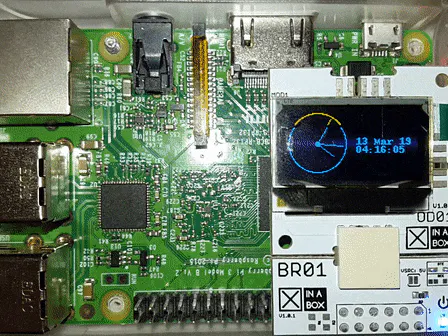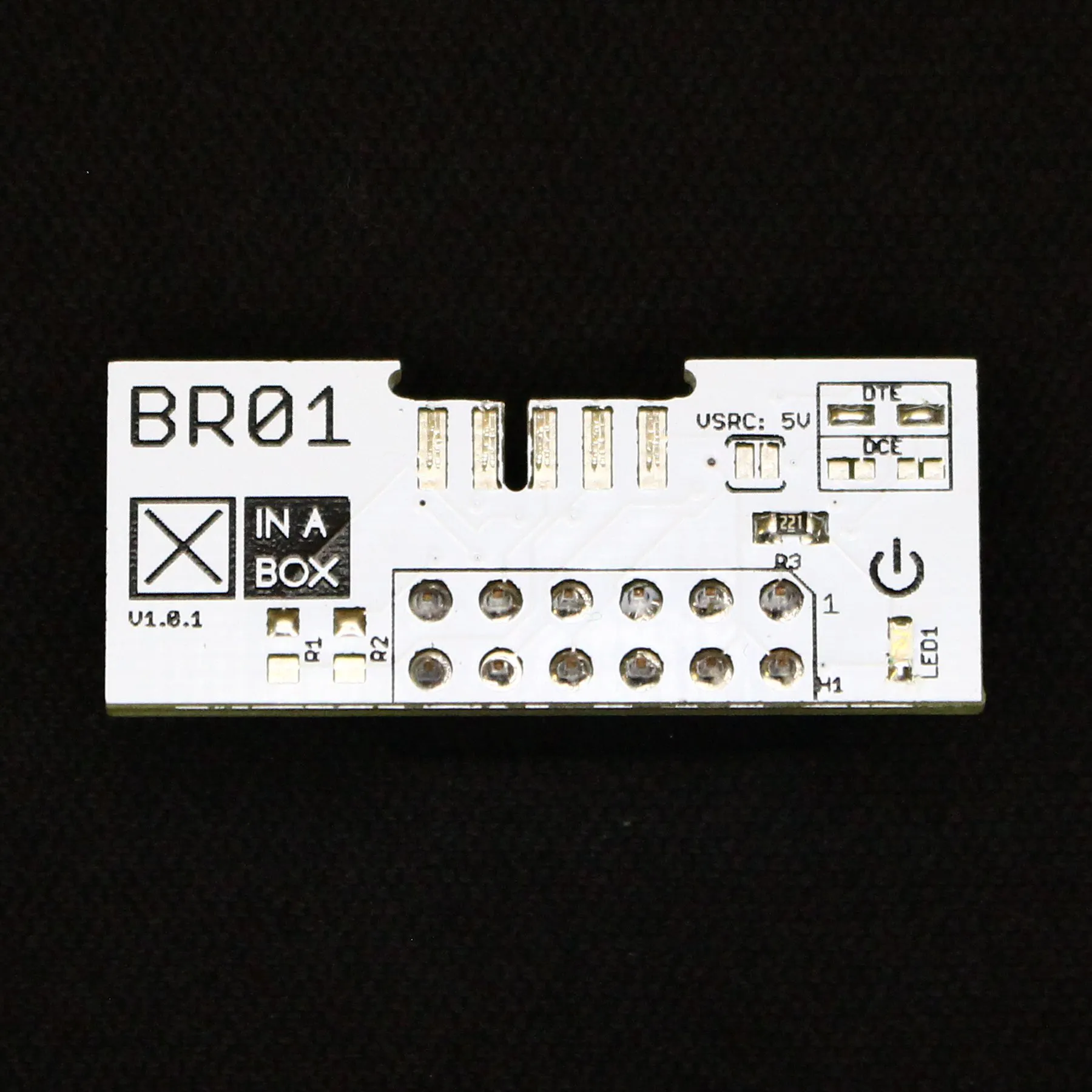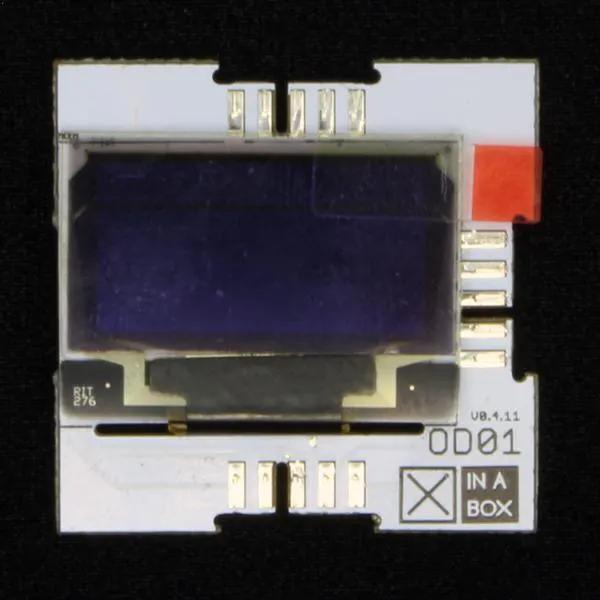# Clock Display with XinaBox OD01 and Raspberry Pi

Learn how to display the current time with XinaBox xChip OD01 and Raspberry Pi.

EasyFull instructions provided15 minutes209## Things used in this project

### Hardware componentsXinaBox BR01
×1XinaBox OD01
×1Raspberry Pi 3 Model B
×1
 (Optional) HDMI cable
×1

### Software apps and online servicesRaspberry Pi Raspbian

## Code

### Clock.py

Python
Example from luma.oled
```#!/usr/bin/env python

# -*- coding: utf-8 -*-

# Copyright (c) 2014-18 Richard Hull and contributors

# See LICENSE.rst for details.

# PYTHON_ARGCOMPLETE_OK

"""

An analog clockface with date & time.

Ported from:

https://gist.github.com/TheRayTracer/dd12c498e3ecb9b8b47f#file-clock-py

"""

import math

import time

import datetime

from demo_opts import get_device

from luma.core.render import canvas

def posn(angle, arm_length):

dx = int(math.cos(math.radians(angle)) * arm_length)

dy = int(math.sin(math.radians(angle)) * arm_length)

return (dx, dy)

def main():

today_last_time = "Unknown"

while True:

now = datetime.datetime.now()

today_date = now.strftime("%d %b %y")

today_time = now.strftime("%H:%M:%S")

if today_time != today_last_time:

today_last_time = today_time

with canvas(device) as draw:

now = datetime.datetime.now()

today_date = now.strftime("%d %b %y")

margin = 4

cx = 30

cy = min(device.height, 64) / 2

left = cx - cy

right = cx + cy

hrs_angle = 270 + (30 * (now.hour + (now.minute / 60.0)))

hrs = posn(hrs_angle, cy - margin - 7)

min_angle = 270 + (6 * now.minute)

mins = posn(min_angle, cy - margin - 2)

sec_angle = 270 + (6 * now.second)

secs = posn(sec_angle, cy - margin - 2)

draw.ellipse((left + margin, margin, right - margin, min(device.height, 64) - margin), outline="white")

draw.line((cx, cy, cx + hrs, cy + hrs), fill="white")

draw.line((cx, cy, cx + mins, cy + mins), fill="white")

draw.line((cx, cy, cx + secs, cy + secs), fill="red")

draw.ellipse((cx - 2, cy - 2, cx + 2, cy + 2), fill="white", outline="white")

draw.text((2 * (cx + margin), cy - 8), today_date, fill="yellow")

draw.text((2 * (cx + margin), cy), today_time, fill="yellow")

time.sleep(0.1)

if __name__ == "__main__":

try:

device = get_device()

main()

except KeyboardInterrupt:

pass
```

## Credits

### KalbeAbbas

7 projects • 2 followers
An enthusiastic and dedicated Electronic engineer graduated from SSUET Karachi, Pakistan. Loves to discover Embedded electronics projects.
Thanks to XinaBox.